#NUM error in Excel occurs when values in formulas are invalid.

It is mostly shown in cases where there is a problem with numbers i.e. the data type entered is not supported in the argument of the formula.

Once you adjust the number input, the calculation can be performed and the error disappears!

In the article, you will be shown the different reasons for #NUM error in Excel and how to fix it:

### What does #NUM mean in Excel?

It basically means that the calculation cannot be performed due to limitations or errors.

This can be due to the fact that the number is too big or small. It also includes impossible calculations like calculating the square root of a negative number, or if the input for a function is invalid, or if an iterating formula like IRR cannot find the result.

Follow this detailed tutorial on #NUM Excel and download this Excel workbook to practice along and understand better:

### How to fix #NUM error in Excel?

Let’s look into more detail and understand the causes and solutions to the #NUM! Excel.

Example 1 – Number size

Excel has a limitation to the size of the number that it can display. If you enter a number is too large or too small,you will get Excel #NUM Error.

As you can see, in cell C7 we are trying to multiply 5 by itself 500 times. The result will be a really large number that Excel is unable to display. Hence. it returns NUM Excel.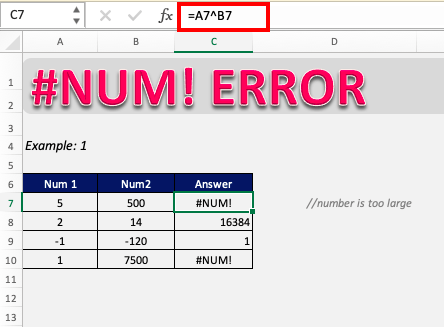In cell C10, we are trying to divide 1 by 10^750. Since this will be a very small number Excel cannot perform calculations and hence returns #NUM error.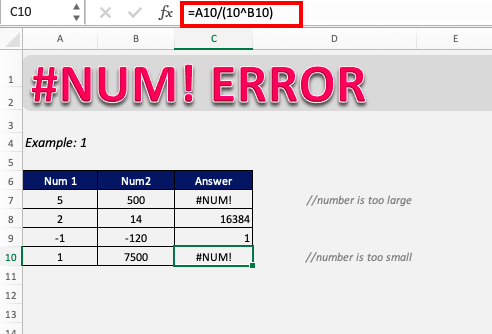Excel is not able to show these values because of its inherent limitations. As shown, other smaller values are calculated and shown in C8 and C9.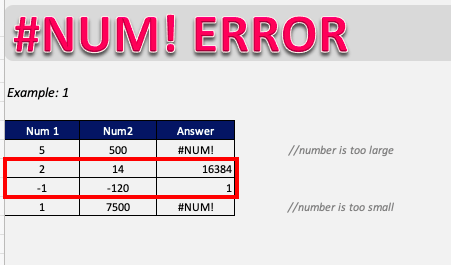Example 2 – Impossible calculations

Excel returns the #NUM! Error when it is faced with a calculation that is impossible to do.

As you can see, the square root or log of the positive numbers has results but the square root or log of the negative numbers is impossible to calculate.

In this example, we are trying to find the square root of -3.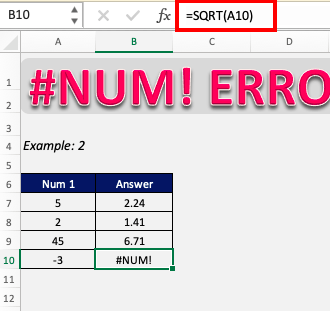Since we are trying to calculate the square root of a negative number, we will get #NUM error in Excel. In this particular case, you can rectify this issue by taking the absolute value of the number.

ABS function returns the absolute value of a number. It converts negative numbers to positive numbers and keeps the positive numbers unaffected.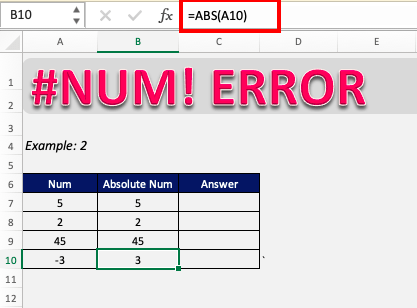Including the ABS function into the formula uses the absolute value of the number in column A, so now Excel can calculate the number and return a valid answer.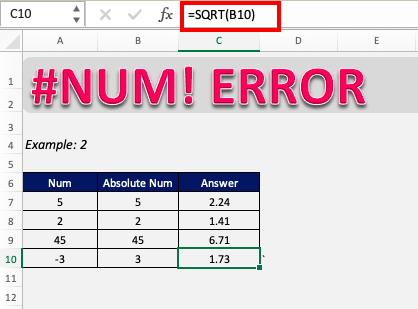Example 3 – IRR function not working

Excel might show the #NUM! error when using IRR function because of either one of the two reasons:

• Cash flow does not contain at least one negative and one positive value.
• Formula can’t find a result.

In this example, Excel results a #NUM error instead of proving a value.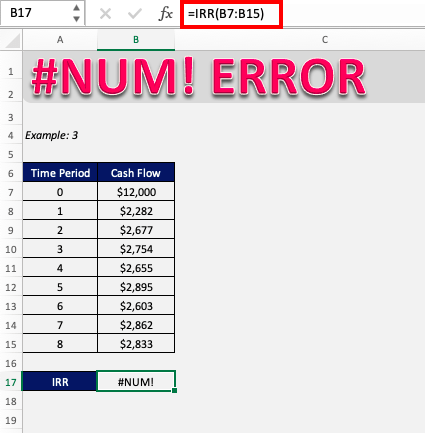This is because the cash flows provided in the range (B7:B15) are positive and IRR requires at least one negative value as the initial cost of business.

Initial payments are provided as negative value indicating outflow of cash and income payments are represented by positive values. Once you have incorporated this, the function will provide the correct result!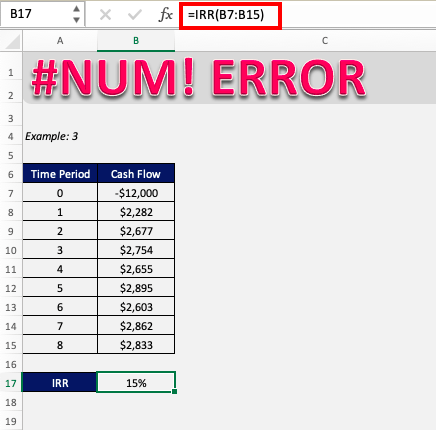IRR function can also display #NUM! error when the function iterates and can’t find a result after several tries.

To overcome this, you can increase the maximum number of iterations that Excel performs to get a result.

To increase the number of iterations:

STEP 1: Go to File > Options > Formulas to arrive at the window below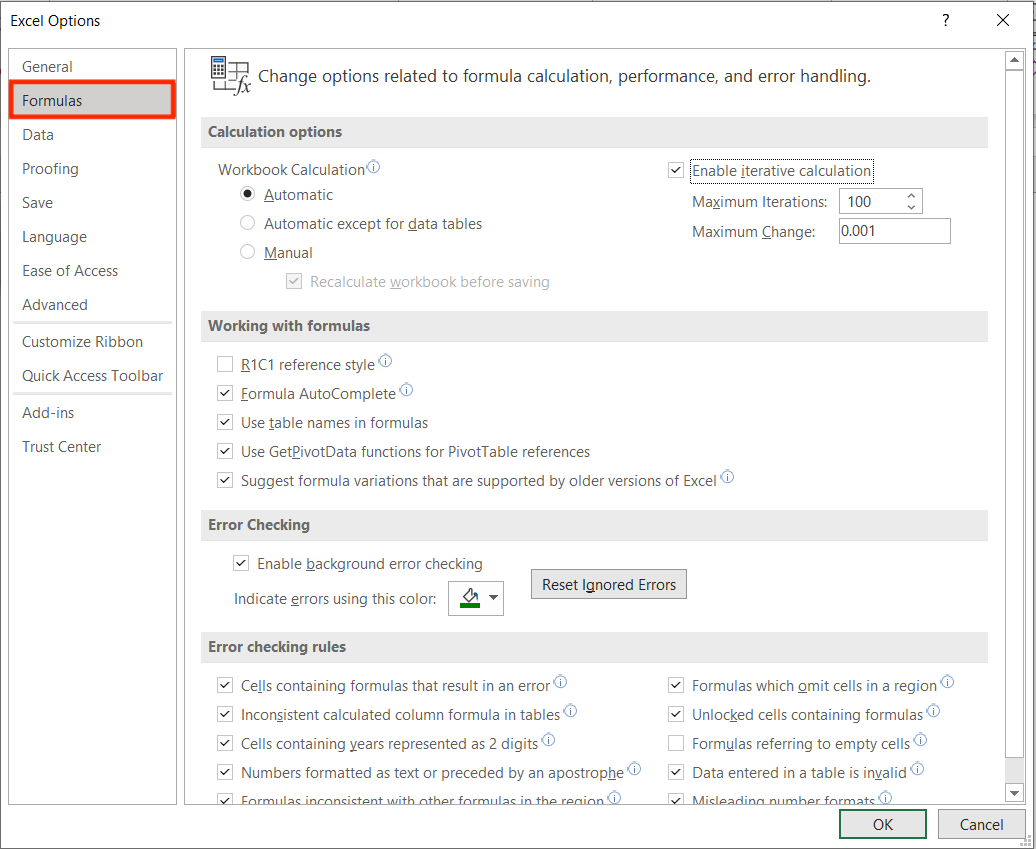STEP 2: Under the Calculation options group, check the Enable iterative calculation box.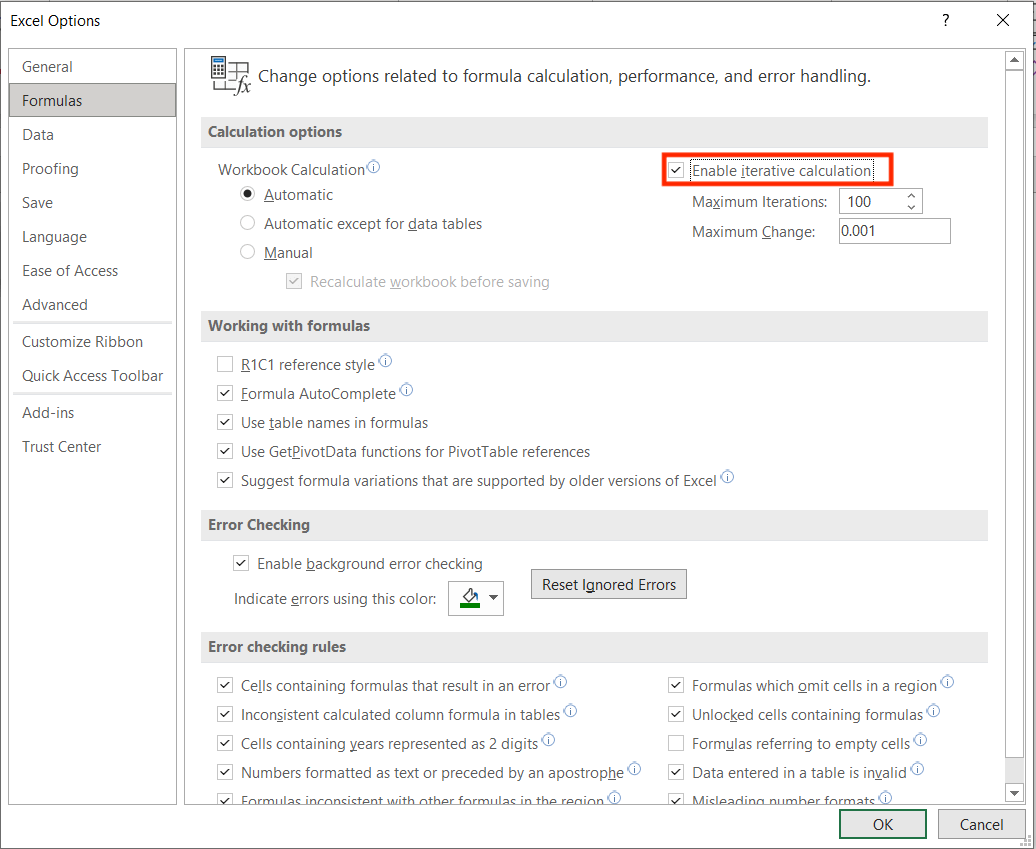STEP 3: In the Maximum Iterations box, type the number of times you want Excel to recalculate.

The higher the number of iterations, the more time Excel needs to calculate a worksheet. Increasing this can help give a result to your calculation.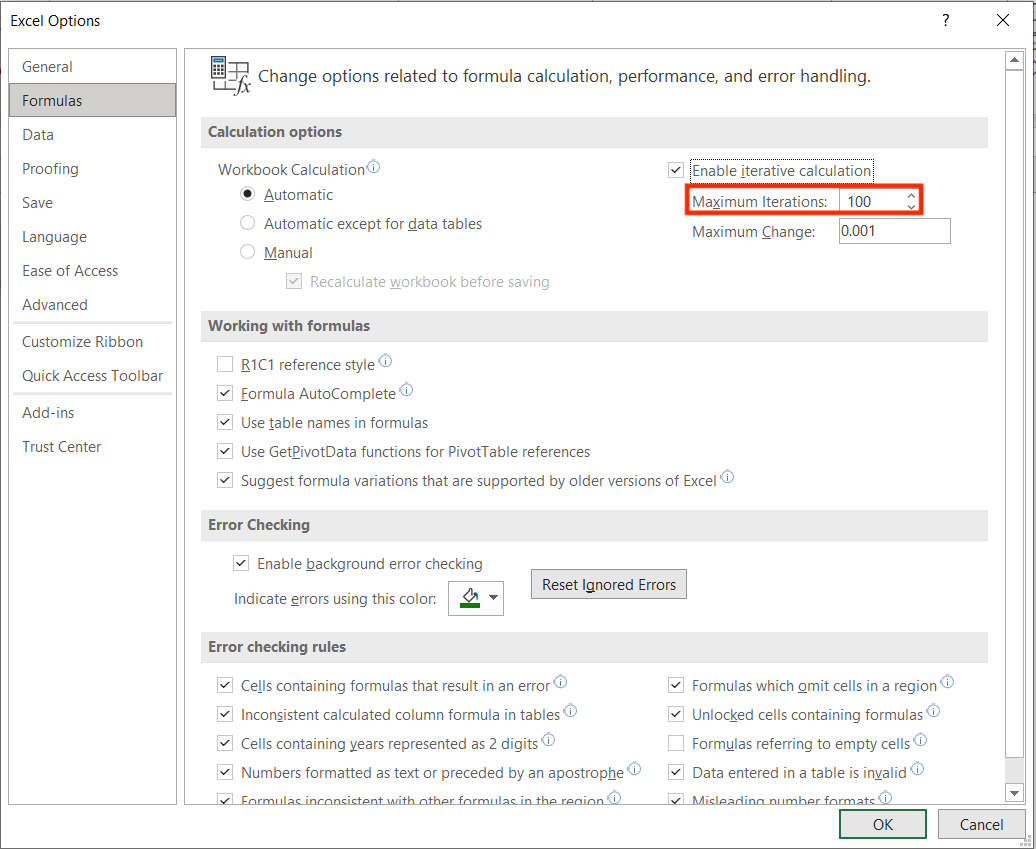STEP 4: In the Maximum Change box, enter the amount of deviation that you are willing to accept between the calculation results.

The smaller the number, the more accurate the result. Increasing this can help give an approximate answer to your calculations.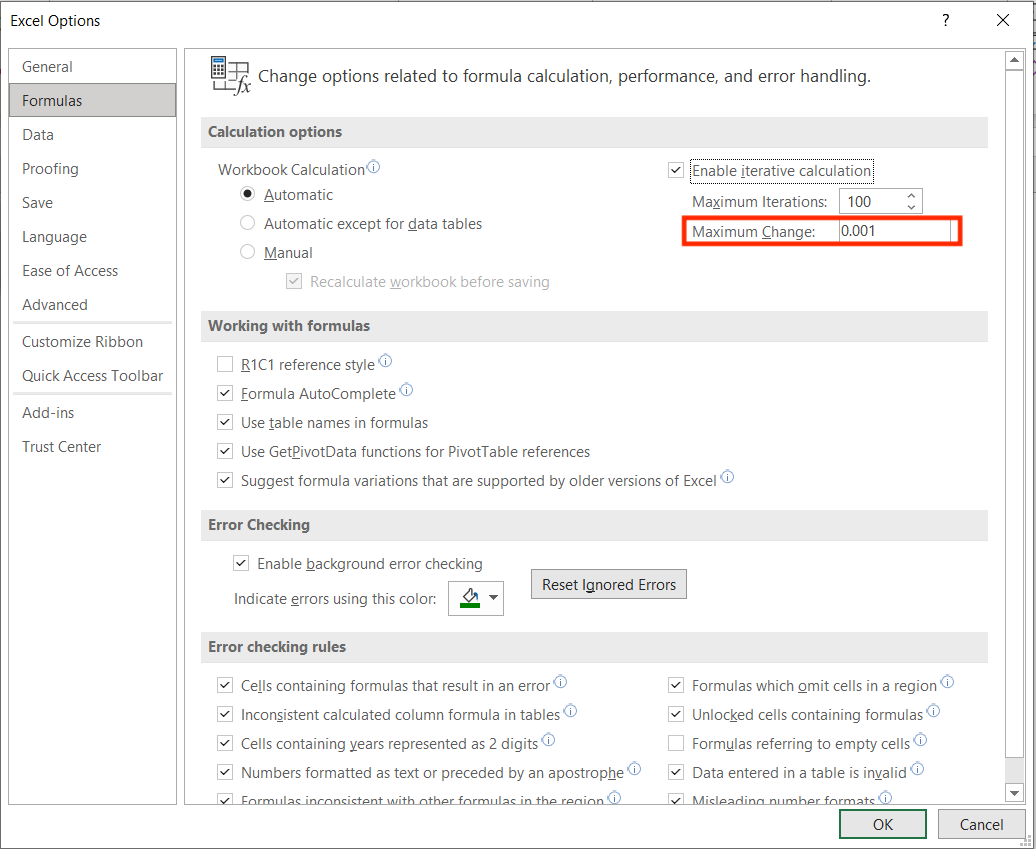### Conclusion

After this exercise, we hope that you now understand why you were experiencing the #NUM error in Excel and that you can now easily rectify and overcome this error and continue with smooth functioning in Excel!